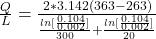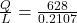## Water flows through a cast steel pipe (k = 50 W m.K, ε = 0.8) with an outer diameter of 104mm and 2 mm wall thickness. Calculate the heat lo

Question

Water flows through a cast steel pipe (k = 50 W m.K, ε = 0.8) with an outer diameter of 104mm and 2 mm wall thickness. Calculate the heat loss (convection and radiation) per meter length of uninsulated pipe when the water temperature is 90 oC, the outside air temperature is -10 oC, the water side heat transfer coefficient is 300 W m2.K and theoutside heat transfer coefficient is 20 W/m^2K.

in progress 0
6 months 2021-07-22T02:00:06+00:00 1 Answers 185 views 0

## Answers ( )

The heat loss per unit length is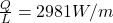Explanation:

From the question we are told that

The outer diameter of the pipe is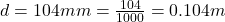The thickness is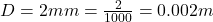The temperature  of water is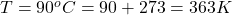The outside air temperature is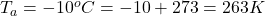The water side heat transfer coefficient is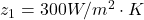The  heat transfer coefficient is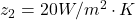The heat lost per unit length is mathematically represented as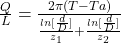Substituting values